# 2011 A-level H2 Mathematics (9740) Paper 2 Question 12 Suggested SolutionsAll solutions here are SUGGESTED. Mr. Teng will hold no liability for any errors. Comments are entirely personal opinions.

(i)
Let X denote the number of people joining the queue in 4 minutes.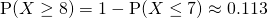(ii)
Let Y denotes number of people joining the queue in t seconds.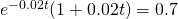Using the Graphing Calculator, t = 55

(iii)
Let W denote the number of people joining the queue in 15 minutes.Since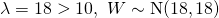approximately.

Let V denote the number of people leaving the queue in 15 minutes.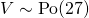Sinceapproximately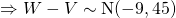approximatelyby continuity correction(iv)
The average number of people joining the queue per minute may not remain constant throughout a period of long hours as they have different traveling time. There might be no flight departing too. Thus, it would not appropriate.

Not readable? Change text.